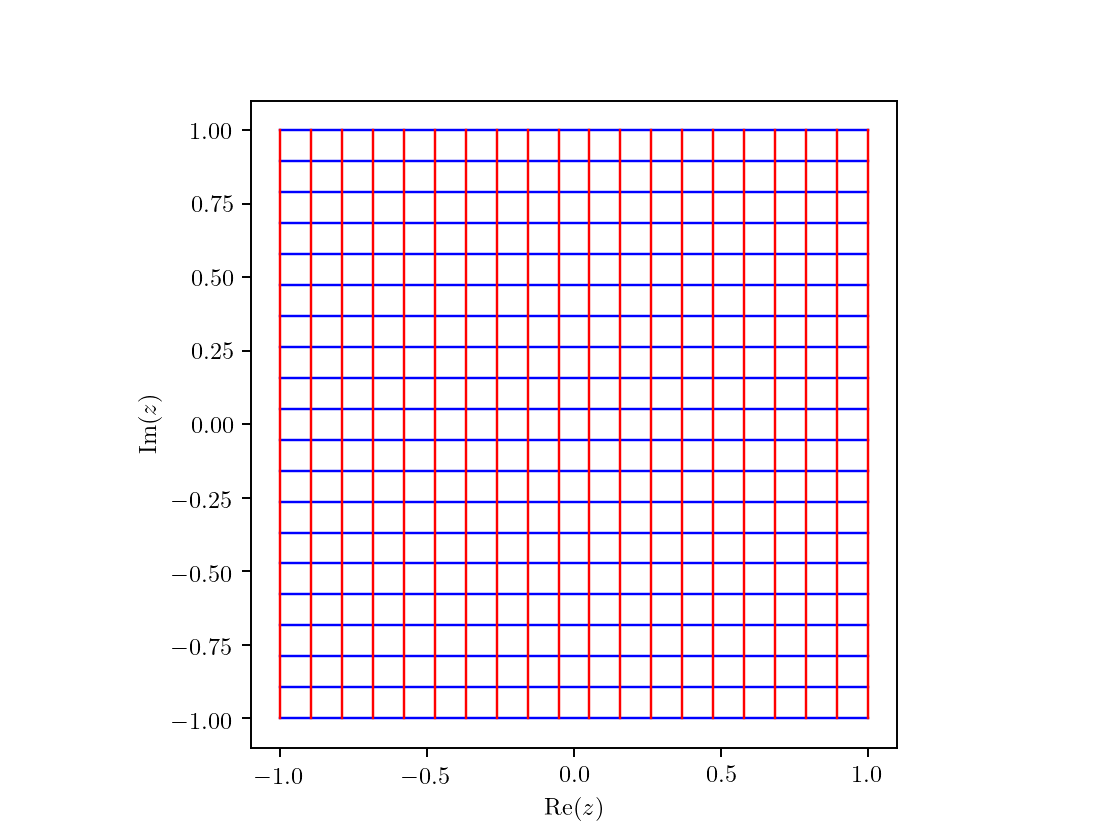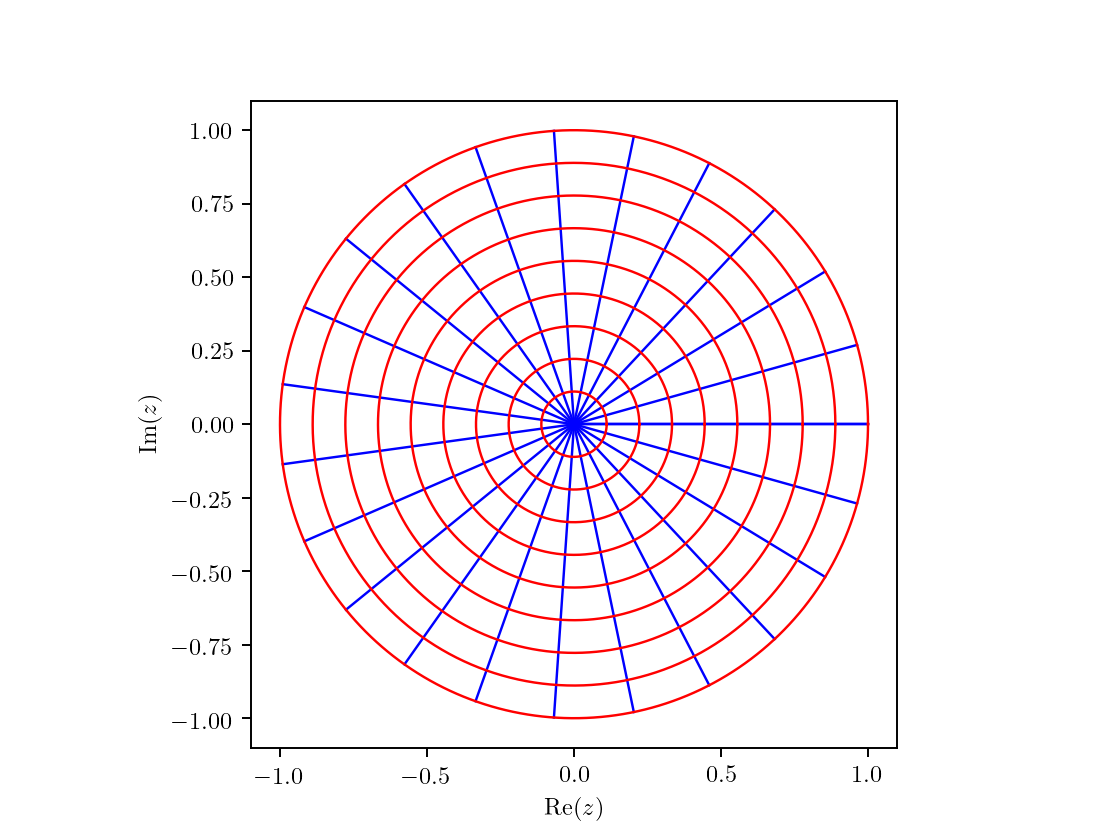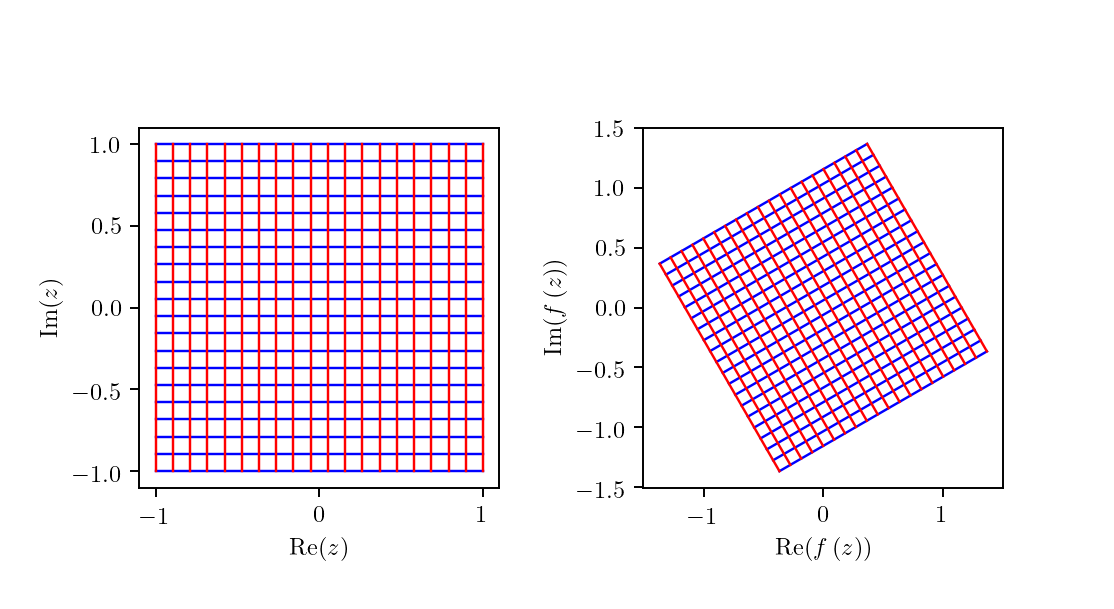# Complex Mappings¶

A visualisation of a few examples of complex mappings via a simple python API.

In :
%matplotlib notebook
%run api.py

# constants
PI = np.pi
TAU = 2 * PI
HALF_PI = PI / 2


The API provides the functions:

• init_grid: creates complex gridlines points
• init_polar: creates complex polar coordinate points
• plot_map: plots color-coded curves in the complex plane
In :
grid = init_grid()
plot_map(grid, map=None)Out:
<matplotlib.axes._subplots.AxesSubplot at 0x7fd4ed947d90>
In :
polar = init_polar()
plot_map(polar, map=None)Out:
<matplotlib.axes._subplots.AxesSubplot at 0x7fd4ea9b0890>

## Mappings¶

In this section we will explore a few examples of complex mappings. Throughout this notebook we will use the following notation:

• $z = x + i y$ where $x$ and $y$ are real variables
• $w = f(z) = u(x,y) + i v(x,y)$ where $u$ and $v$ are real-valued functions

For polar coordinates:

• $z = r\cdot e^{i\theta}$ where $r$ and $\theta$ are real variables
• $w = f(z) = \rho(r,\theta)\cdot e^{i \varphi(r,\theta)}$ where $\rho$ and $\varphi$ are real-valued functions

### Rotation¶

The rotation by an angle $\alpha$ is defined by the transformation $$f(z) = z\cdot e^{i\alpha}$$

Which can be easily deduced from the polar form $f(z) = r\cdot e^{i(\theta+\alpha)} \rightarrow\begin{cases}\rho=r\\ \varphi=\theta+\alpha\end{cases}$

Let's see how the cartesian grid is transformed for $\alpha=\frac{\pi}{6}$

In :
rotation = lambda z : z * np.exp(1j * PI / 6)
plot_map(grid, rotation)Out:
(<matplotlib.axes._subplots.AxesSubplot at 0x7fd4ea1f7490>,
<matplotlib.axes._subplots.AxesSubplot at 0x7fd4e99e5fd0>)
In :
plot_map(polar, rotation, color_each=True)